# Automatic Parking Valet with Unreal Engine Simulation

This example shows the design of a hybrid controller for an automatic searching and parking task. You will learn how to combine adaptive model predictive control (MPC) with reinforcement learning (RL) to perform a parking maneuver. The path of the vehicle is visualized using the Unreal Engine® simulation environment.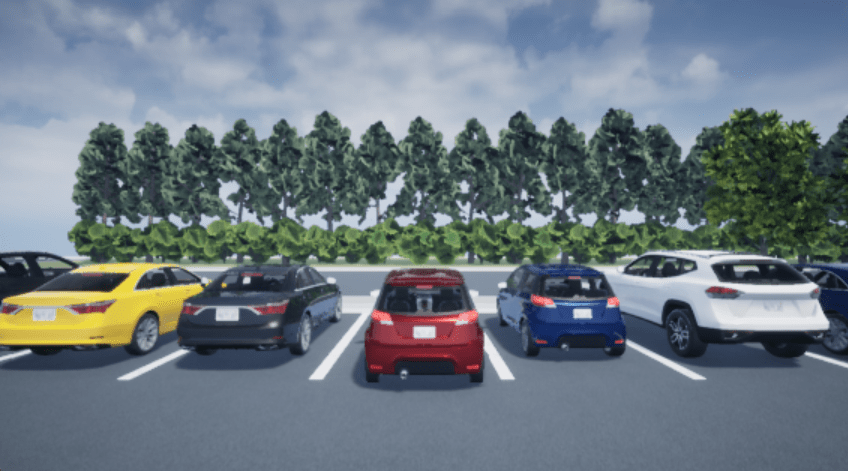The control objective is to park the vehicle in an empty spot after starting from an initial pose. The control algorithm executes a series of maneuvers while sensing and avoiding obstacles in tight spaces. It switches between an adaptive MPC controller and a reinforcement learning agent to complete the parking maneuver. The adaptive MPC controller moves the vehicle at a constant speed along a reference path while searching for an empty parking spot. When a spot is found, the reinforcement learning agent takes over and executes a pretrained parking maneuver. Prior knowledge of the environment (the parking lot) including the locations of the empty spots and parked vehicles is available to both the controllers.

### Parking Lot

The parking lot environment used in this example is a subsection of the Large Parking Lot (Automated Driving Toolbox) scene. The parking lot is represented by the `ParkingLotEnvironment` object, which stores information on the ego vehicle, empty parking spots, and static obstacles (parked cars and boundaries). Each parking spot has a unique index number and an indicator light that is either green (free) or red (occupied). Parked vehicles are represented in black while other boundaries are outlined in green.Specify a sample time ${\mathit{T}}_{\mathit{s}}$ (seconds) for the controllers and a simulation time ${\mathit{T}}_{\mathit{f}}$ (seconds).

```Ts = 0.1; Tf = 50;```

Create a reference path for the ego vehicle using the `createReferenceTrajectory` helper function included with this example. The reference path starts from the south-east corner of the parking lot and ends in the west as displayed with the dashed pink line.

`xRef = createReferenceTrajectory(Ts,Tf);`

Create a `ParkingLotEnvironment` object with a free spot at index 32 and the specified reference path `xRef`.

```freeSpotIndex = 32; map = ParkingLotEnvironment(freeSpotIndex,"Route",xRef);```

Specify an initial pose $\left({\mathit{X}}_{0},{\mathit{Y}}_{0},{\theta }_{0}\right)$ for the ego vehicle. The units of ${\mathit{X}}_{0}$ and ${\mathit{Y}}_{0}$ are meters and ${\theta }_{0}$ is in radians.

`egoInitialPose = [40 -55 pi/2];`

Compute the target pose for the vehicle using the `createTargetPose` function. The target pose corresponds to the location in `freeSpotIdx`.

`egoTargetPose = createTargetPose(map,freeSpotIndex);`

### Sensor Modules

The parking algorithm uses geometric approximations of a camera and a lidar sensor to gather information from the parking lot environment.

#### Camera

In this example, the field of view of a camera mounted on the ego vehicle is represented by the area shaded in green in the following figure. The camera has a field of view $\phi$ bounded by $±\pi /3$ radians and a maximum measurement depth ${\mathit{d}}_{\mathrm{max}}$ of 10 m. As the ego vehicle moves forward, the camera module senses the parking spots within the field of view and determines whether a spot is free or occupied. For simplicity, this action is implemented using geometrical relationships between the spot locations and the current vehicle pose. A parking spot is within the camera range if ${\mathit{d}}_{\mathit{i}}\le {\mathit{d}}_{\mathrm{max}}$ and ${\phi }_{\mathrm{min}}\le {\phi }_{\mathit{i}}\le {\phi }_{\mathrm{max}}$, where ${\mathit{d}}_{\mathit{i}}$ is the distance to the parking spot and ${\phi }_{\mathit{i}}$ is the angle to the parking spot.#### Lidar

The lidar sensor in this example is modeled using radial line segments emerging from the geometric center of the vehicle. Distances to obstacles are measured along these line segments. The maximum measurable lidar distance along any line segment is 6 m. The reinforcement learning agent uses these readings to determine the proximity of the ego vehicle to other vehicles in the environment.### Auto Parking Valet Model

Load the auto parking valet parameters.

`autoParkingValetParams3D`

The parking valet model, including the controllers, ego vehicle, sensors, and parking lot, is implemented in a Simulink model. Open the model.

```mdl = "rlAutoParkingValet3D"; open_system(mdl)```In this model:

• The vehicle is modeled in the Ego Vehicle Model subsystem. The kinematics is represented by a single-track bicycle kinematics model with two input signals: vehicle speed $\mathit{v}$ (m/s) and steering angle $\delta$ (radians).

• The adaptive MPC and RL agent blocks are found in the MPC Tracking Controller and RL Controller subsystems, respectively.

• Mode switching between the controllers is handled by the Vehicle Mode subsystem, which outputs Search and Park signals. Initially, the vehicle is in search mode and the adaptive MPC controller tracks the reference path. When a free spot is found, the Park signal activates the RL Agent to perform the parking maneuver.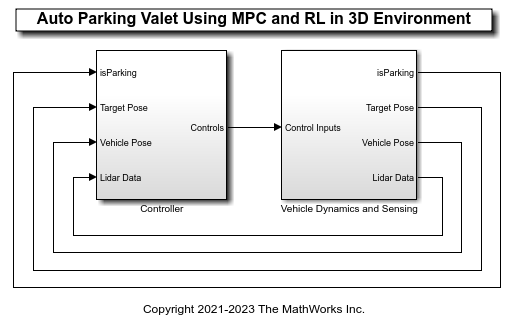• The Visualization Subsystem handles animation of the environment. Double-click this subsystem to specify the visualization options.To plot the environment in a figure, set the 2D Visualization parameter to `On`.

If you have Automated Driving Toolbox™ software installed, you can set the Unreal Engine Visualization parameter to `On` to display the vehicle animation in the Unreal Engine environment. Enabling the Unreal Engine simulation can degrade simulation performance. Therefore, set the parameter to `Off` when training the agent.

### Adaptive Model Predictive Controller Design

Create the adaptive MPC controller object for reference trajectory tracking using the `createMPCForParking` script. For more information on adaptive MPC, see Adaptive MPC (Model Predictive Control Toolbox).

`createMPCForParking3D;`

### Reinforcement Learning Controller Design

To train the reinforcement learning agent, you must create an environment interface and an agent object.

#### Create Environment

The environment for training is the region shaded in red in the following figure. Due to symmetry in the parking lot, training within this region is sufficient for the policy to adjust to other regions after coordinate transformations are applied to the observations. Constraining the training to this region also significantly reduces training duration when compared to training over the entire parking lot space.For this environment:

• The training region is a 13.625 m x 12.34 m space with the target spot at its horizontal center.

• The observations are the position errors ${\mathit{X}}_{\mathit{e}}$ and ${\mathit{Y}}_{\mathit{e}}$ of the ego vehicle with respect to the target pose, the sine and cosine of the true heading angle $\theta$, and the lidar sensor readings.

• The vehicle speed during parking is a constant 2 m/s.

• The action signals are discrete steering angles that range between +/- $\pi /4$ radians in steps of 0.2618 radians or 15 degrees.

• The vehicle is considered parked if the errors with respect to target pose are within specified tolerances of +/- 0.75 m (position) and +/-10 degrees (orientation).

• The episode terminates if the ego vehicle goes out of the bounds of the training region, collides with an obstacle, or parks successfully.

• The reward ${\mathit{r}}_{\mathit{t}}$ provided at time t, is:

`${\mathit{r}}_{\mathit{t}}=2{\mathit{e}}^{-\left(0.05{{\mathit{X}}_{\mathit{e}}}^{2}+0.04{{\mathit{Y}}_{\mathit{e}}}^{2}\right)}+0.5{\mathit{e}}^{-40{{\theta }_{\mathit{e}}}^{2}}-0.05{\delta }^{2}+100{\mathit{f}}_{\mathit{t}}-50{\mathit{g}}_{\mathit{t}}$`

Here, ${\mathit{X}}_{\mathit{e}}$, ${\mathit{Y}}_{\mathit{e}}$, and ${\theta }_{\mathit{e}}$ are the position and heading angle errors of the ego vehicle from the target pose, while $\delta$ is the steering angle. ${\mathit{f}}_{\mathit{t}}$ (0 or 1) indicates whether the vehicle has parked and ${\mathit{g}}_{\mathit{t}}$ (0 or 1) indicates if the vehicle has collided with an obstacle or left the training region at time $\mathit{t}$.

The coordinate transformations on vehicle pose $\left(\mathit{X},\mathit{Y},\theta \right)$ observations for different parking spot locations are as follows:

• Parking spots 1-14: $\stackrel{‾}{\mathit{X}}=\mathit{X},\stackrel{‾}{\mathit{Y}}=\mathit{Y}+20.41,\text{\hspace{0.17em}}\stackrel{‾}{\theta }=\theta$

• Parking spots 15-28: $\stackrel{‾}{\mathit{X}}=41-\mathit{X},\stackrel{‾}{\mathit{Y}}=-64.485-\mathit{Y},\text{\hspace{0.17em}}\stackrel{‾}{\theta }=\theta -\pi$

• Parking spots 29-37: $\mathrm{no}\text{\hspace{0.17em}}\mathrm{transformation}$

• Parking spots38-46: $\stackrel{‾}{\mathit{X}}=41-\mathit{X},\stackrel{‾}{\mathit{Y}}=-84.48-Y,\stackrel{‾}{\text{\hspace{0.17em}}\theta }=\theta -\pi$

Create the observation and action specifications for the environment.

```nObs = 16; nAct = 1; obsInfo = rlNumericSpec([nObs 1]); obsInfo.Name = "observations"; actInfo = rlNumericSpec([nAct 1],LowerLimit=-1,UpperLimit=1); actInfo.Name = "actions";```

Create the Simulink environment interface, specifying the path to the RL Agent block.

```blk = mdl + "/Controller/RL Controller/RL Agent"; env = rlSimulinkEnv(mdl,blk,obsInfo,actInfo);```

Specify a reset function for training. The `autoParkingValetResetFcn` function resets the initial pose of the ego vehicle to random values at the start of each episode.

`env.ResetFcn = @autoParkingValetResetFcn3D;`

For more information on creating Simulink environments, see `rlSimulinkEnv`.

#### Create Agent

The agent in this example is a twin-delayed deep deterministic policy gradient (TD3) agent. TD3 agents rely on actor and critic approximator objects to learn the optimal policy. To learn more about TD3 agents, see Twin-Delayed Deep Deterministic (TD3) Policy Gradient Agents.

The actor and critic networks are initialized randomly. Ensure reproducibility by fixing the seed of the random generator.

`rng(0)`

TD3 agents use two parametrized Q-value function approximators to estimate the value of the policy. To model the parametrized Q-value function within both critics, use a neural network with 16 inputs and one output. The output of the critic network is the state-action value function for a taking a given action from a given observation.

Define each network path as an array of layer objects. Assign names to the input and output layers of each path. These names allow you to connect the paths and then later explicitly associate the network input and output layers with the appropriate environment channel.

```% Main path mainPath = [ featureInputLayer(nObs,Name="StateInLyr") fullyConnectedLayer(128) concatenationLayer(1,2,Name="concat") reluLayer fullyConnectedLayer(128) reluLayer fullyConnectedLayer(1,Name="CriticOutLyr") ]; % Action path actionPath = [ featureInputLayer(nAct,Name="ActionInLyr") fullyConnectedLayer(128,Name="fc2") ]; % Convert to layergraph object and connect layers criticNet = layerGraph(mainPath); criticNet = addLayers(criticNet, actionPath); criticNet = connectLayers(criticNet,"fc2","concat/in2");```

Convert to a `dlnetwork` object and display the number of learnable parameters.

```criticdlnet = dlnetwork(criticNet); summary(criticdlnet)```
``` Initialized: true Number of learnables: 35.4k Inputs: 1 'StateInLyr' 16 features 2 'ActionInLyr' 1 features ```

Create the critics using `criticdlnet`, the environment observation and action specifications, and the names of the network input layers to be connected with the environment observation and action channels. For more information see `rlQValueFunction`.

```critic1 = rlQValueFunction(criticNet,obsInfo,actInfo,... ObservationInputNames="StateInLyr",ActionInputNames="ActionInLyr"); critic2 = rlQValueFunction(criticNet,obsInfo,actInfo,... ObservationInputNames="StateInLyr",ActionInputNames="ActionInLyr");```

Create the neural network for the actor. The output of the actor network is the steering angle.

```anet = [ featureInputLayer(nObs) fullyConnectedLayer(128) reluLayer fullyConnectedLayer(128) reluLayer fullyConnectedLayer(nAct) tanhLayer ]; actorNet = layerGraph(anet);```

Convert to a `dlnetwork` object and display the number of learnable parameters.

```actordlnet = dlnetwork(actorNet); summary(actordlnet)```
``` Initialized: true Number of learnables: 18.8k Inputs: 1 'input' 16 features ```

Create the actor object for the TD3 agent. For more information see `rlContinuousDeterministicActor`.

`actor = rlContinuousDeterministicActor(actordlnet,obsInfo,actInfo);`

Specify the agent options and create the TD3 agent. For more information on TD3 agent options, see `rlTD3AgentOptions`.

```agentOpts = rlTD3AgentOptions(SampleTime=Ts, ... DiscountFactor=0.99, ... ExperienceBufferLength=1e6, ... MiniBatchSize=128);```

Set the noise options for exploration.

```agentOpts.ExplorationModel.StandardDeviation = 0.1; agentOpts.ExplorationModel.StandardDeviationDecayRate = 1e-4; agentOpts.ExplorationModel.StandardDeviationMin = 0.01;```

For this example, set the actor and critic learn rates to `1e-3` and `2e-3`, respectively. Set a gradient threshold factor of `1` to limit the gradients during training. For more information, see `rlOptimizerOptions`.

Specify training options for the actor.

```agentOpts.ActorOptimizerOptions.LearnRate = 1e-3; agentOpts.ActorOptimizerOptions.GradientThreshold = 1; agentOpts.ActorOptimizerOptions.L2RegularizationFactor = 1e-3;```

Specify training options for the critic.

```agentOpts.CriticOptimizerOptions(1).LearnRate = 2e-3; agentOpts.CriticOptimizerOptions(2).LearnRate = 2e-3; agentOpts.CriticOptimizerOptions(1).GradientThreshold = 1; agentOpts.CriticOptimizerOptions(2).GradientThreshold = 1;```

Create the agent using the actor, the critics, and the agent options objects. For more information, see `rlTD3Agent`.

`agent = rlTD3Agent(actor,[critic1 critic2], agentOpts);`

#### Train Agent

To train the agent first specify the training options.

The agent is trained for a maximum of `10000` episodes with each episode lasting a maximum of `200` time steps. The training terminates when the maximum number of episodes is reached or the average reward over `200` episodes reaches the value of `120` or more. Specify the options for training using the `rlTrainingOptions` function.

```trainOpts = rlTrainingOptions(... MaxEpisodes=10000,... MaxStepsPerEpisode=200,... ScoreAveragingWindowLength=200,... Plots="training-progress",... StopTrainingCriteria="AverageReward",... StopTrainingValue=120);```

Train the agent using the `train` function. Fully training this agent is a computationally intensive process that may take several hours to complete. To save time while running this example, load a pretrained agent by setting `doTraining` to `false`. To train the agent yourself, set `doTraining` to `true`.

```doTraining = false; if doTraining trainingResult = train(agent,env,trainOpts); else load("rlAutoParkingValetAgent.mat","agent"); end```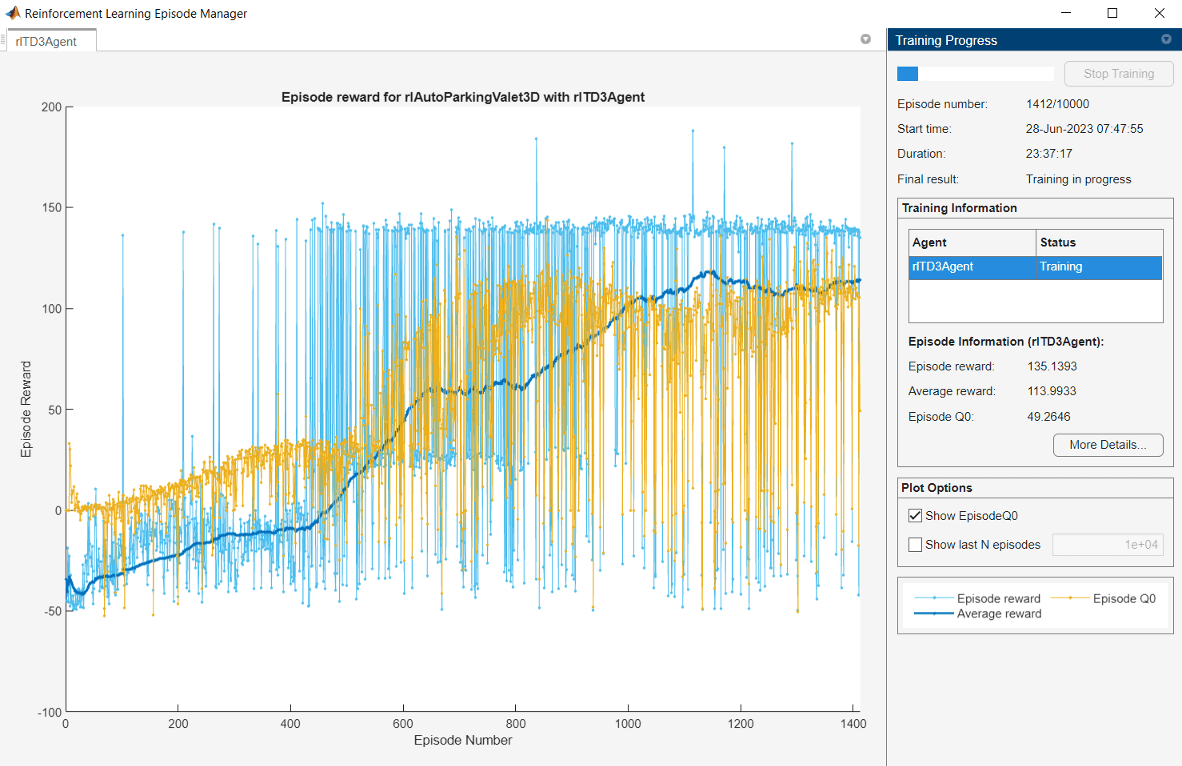To Validate the trained agent, simulate the model and observe the parking maneuver.

`sim(mdl);`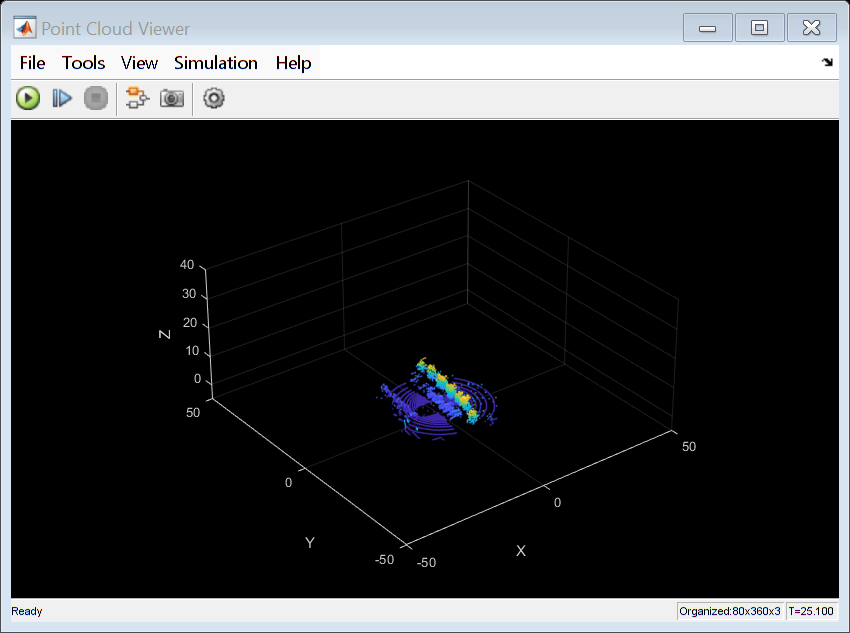The vehicle tracks the reference path using the MPC controller before switching to the RL controller when the target spot is detected. The vehicle then completes the parking maneuver.

To view the trajectory, open the Ego Vehicle Pose scope.

`open_system(mdl + "/Ego Vehicle Model/Ego Vehicle Pose")`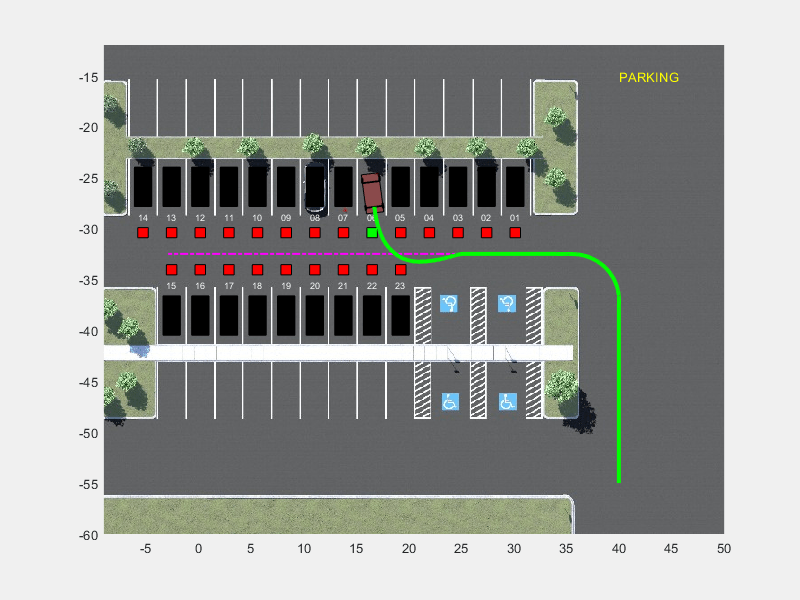#### Unreal Engine Simulation

Turn on the Unreal Engine visualization by opening the Visualization block and setting the Unreal Engine Visualization parameter to `On`. Initializing the Unreal Engine simulation environment can take a few seconds..Park the vehicle in a different spot.

```freeSpotIndex = 20; sim(mdl)```